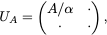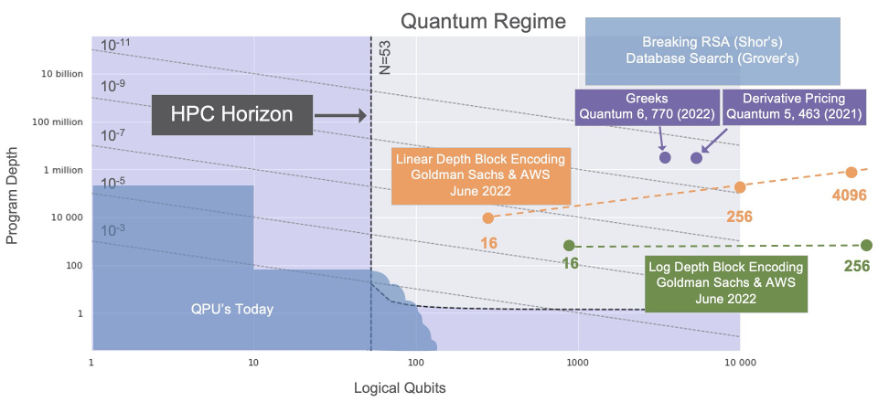# Goldman Sachs and AWS examine efficient ways to load data into quantum computers

by Grant Salton, Mario Berta, Alexander Dalzell, Dave Clader, Nikitas Stamatopoulos, and William Zeng | on | in | Permalink |  Share

Suppose you are trying to solve a problem of interest (e.g., portfolio optimization or machine learning), and you are given some classical data, neatly arranged into a matrix A. Your plan is to solve this problem using a quantum algorithm to process the data. For example, you may be trying to solve a linear system of equations as a subroutine for portfolio optimization. The quantum algorithm is well defined, and you know how to process and interpret the results the quantum computer gives, but now there’s a question: how do you load your classical data into the quantum computer for use by the quantum algorithm? Moreover, how do you do this efficiently if the quantum algorithm will need repeated access to the data?

Schemes for efficiently accessing classical data that has been loaded into quantum memory have been proposed, including a particular scheme known as “block-encoding” of the data. To load data using a block-encoding, one uses special purpose data structures, such as a quantum version of a random access memory — QRAM for short. Block-encodings are ubiquitous in quantum algorithms, but they are an often-overlooked source of overhead when assessing the computational resources needed to run the quantum algorithm. Moreover, concrete implementations of block-encodings have not been explored in depth at the level of quantum circuits. Together with Goldman Sachs, the Amazon Quantum Solutions Lab (QSL) and the AWS Center for Quantum Computing (CQC) filled in some of the missing pieces in a new paper titled “Quantum Resources Required to Block-Encode a Matrix of Classical Data”.

We performed a careful analysis into methods of encoding classical data into quantum states using the well-known block-encoding framework, and we provided realistic estimates of the quantum resources that one would require. Our work was presented at the Quantum Resource Estimation 2022 conference in New York (colocated with the International Symposium on Computer Architecture (ISCA) 2022). Our work allows for practical assessments of the end-to-end computational cost of solving real-world problems on quantum hardware, taking into account the resources required for building these data access structures, and going beyond simple asymptotic computational complexities for the quantum processing unit alone.

In this post, we summarize for you our current understanding of what it takes to load generic data into a quantum computer.

## The Goldman Sachs and AWS collaboration

The Research and Development team at Goldman Sachs works to develop an edge for clients when it comes to technology in finance. Motivated by the theoretical potential of quantum computing, Goldman Sachs has been studying what it will take to make these theoretical advantages practical, often by taking well-defined benchmark problems in finance and estimating the performance specifications that a quantum computer will need to beat to show advantage. Similarly, the QSL works closely with customers to find the best solutions to their most challenging problems (be they quantum, classical, or hybrid), and scientists at the AWS CQC are working to push the state-of-the-art in quantum technologies. Goldman Sachs, the QSL, and the CQC joined forces to evaluate different applications of quantum computers to real-world financial problems. Through a series of collaborative research engagements, Goldman Sachs and the quantum computing team at AWS systematically worked to find suitable use-cases for quantum computers, and appropriate quantum solutions. As financial problems often use large amounts of data, we identified that it would be important to specifically study the resources required for data loading.

## Block-encoding of classical data

As mentioned in the introduction, loading classical data is a crucial component of many quantum algorithms applied to classical computational problems. How, then, do we build a QRAM to store the classical data? These questions have been addressed in several ways by the quantum algorithms community, and, as mentioned earlier, the most common method of encoding the data is known as “block-encoding”. A challenge one faces is that classical data can come in all shapes and sizes, whereas operations in quantum computing are required to have a specific structure (in particular, they must be unitary). Block-encodings address this issue by encoding the matrix A as a block of a larger (unitary) matrix UA with the property thatwhere α is a constant, and the dots indicate unspecified entries that ensure the matrix UA is unitary. In other words, the classical matrix A is encoded as a submatrix in a larger block matrix — the unitary UA.

The block-encoding concept is appealing because the resource cost of quantum algorithms can be expressed in terms of the number of times UA must be used, while the cost of implementing UA can be counted separately. While block-encodings are a staple of many quantum algorithms, something lacking in the literature was a concrete prescription practitioners could use to implement a block-encoding in detail, along with the quantum resources required. In this joint work from AWS and Goldman Sachs, we fill in these gaps and give comprehensive resource estimates accounting for all steps in the block-encoding process. Our findings provide exact resource expressions that had not been previously worked out, and they reflect asymptotic improvements over prior work due to conceptual advancements to the block-encoding implementation. This work provides scientists and engineers with a more complete view of the computational resources required to implement a quantum algorithm, without sweeping anything under the rug.

## Findings on the quantum computational resource requirements

Usually we don’t need to load data into a quantum computer with exact precision; it often suffices to load the data up to some error ε. The quantum resources required to block-encode a classical N×N matrix A (e.g. a matrix of N2 floating point numbers) to precision ε ∈ [0,1) are summarized in the following table. We focus on three main quantum resources that are relevant for a fault-tolerant quantum computation:

1. the amount of quantum memory needed, measured as the number of logical qubits required,
2. the number of a certain type of quantum operation called T-gates that need to be run sequentially, labeled as T-Depth, and
3. the total number of T-gates that the program needs, labeled as T-Count.

T-gates are a computational primitive in the most commonly used model of large-scale quantum computation, and quantum operations are often compiled down into a set of easy-to-perform gates, and a number of difficult-to-perform T-gates. T-gates are computationally expensive, and many architecture proposals for fault-tolerant quantum computers have runtimes that are dominated by T-gates.

 Resource Minimum Depth Minimum Count # Qubits 4N 2 N log (1/ε) T-Depth 10 log N + 24 log (1/ε) 8N + 12 log N (log (1/ε))2 T-Count 12N 2 log (1/ε) 16N log (1/ε) + 12 log N (log (1/ε))2
Table 1: Quantum resources required to block-encode a classical N×N matrix to precision ε. In the table above, we consider two schemes for encoding (shown in the two columns): one that minimizes the T-Depth of the circuit implementation, and one that minimizes the T-Count. We have suppressed small, subleading terms for ease of presentation; interested readers are referred to the paper for a more careful accounting.

Previous analyses had asserted that the minimum T-depth construction could scale polynomially in both log(N) and log(1/ε); our results go farther by showing this polynomial is linear in each of log(N) and log(1/ε) separately, and we even report the constant factors in our expression. The scaling analyses shown in Table 1 can be a bit daunting, so to paint a realistic picture, we calculated these resources for matrices of sizes N=16, N=256, and N=4096 in the following table:

 Resource Minimum Depth Minimum Count # Qubits [1×103, 3×105, 7×107] [4×102, 7×103, 1×105] T-Depth [5×102, 7×102, 8×102] [2×104, 7×104, 2×105] T-Count [7×104, 2×107, 7×109] [3×104, 2×105, 2×106]
Table 2: Example quantum resources required for matrices of sizes [16×16, 256×256, 4096×4096] to precision ε=0.01. To implement a minimum-depth block-encoding of a 4096 x 4096 matrix — an object that represents dozens of megabytes of classical data — 70 million qubits would be needed for the QRAM (roughly the same amount of quantum memory as classical memory), but only 800 parallel layers of T gates. It is conceivable that in future hardware architectures, specialized QRAM qubits could lead to lower resource costs than fully fault-tolerant qubits capable of universal quantum computation.

It can be instructive to compare these concrete resource estimates with the resources required for other quantum algorithms. In Figure 1 we highlight the current state-of-the-art of quantum hardware, showing the number of qubits available and the circuit depth one can apply before noise dominates the calculation. The vertical line indicates a rough High Performance Computing (HPC) horizon — e.g. quantum computer specifications that we can simulate classically in a reasonable amount of time. To the right of the HPC horizon is the quantum regime where we can expect to find advantage through quantum algorithms. In the very top right, with large numbers of qubits and large depths, we find the venerable Shor’s factoring algorithm and Grover search. More recently, Goldman Sachs studied quantum algorithms for pricing derivatives and calculating market risk, finding that one needs thousands of qubits and circuit depths in the millions for these algorithms.

Our analysis shows that one needs orders of magnitude smaller circuit depths, yet hundreds or thousands of qubits to implement block-encodings. In other words, loading the classical data is costly in terms of number of qubits needed, but can be done efficiently in terms of the number of operations needed to load the data. While block-encodings are not used to solve a computational problem themselves, they constitute a crucial component of many larger quantum algorithms. Thus, it is helpful to compare the cost of this algorithmic primitive to other known algorithms, since block-encoding will constitute a lower bound on the complexity of larger algorithms that make use of QRAM data structures.Figure 1: The state-of-the-art in quantum algorithms research. The HPC horizon occurs around ~53 qubits, above which classical supercomputers cannot simulate arbitrary quantum computations. In the quantum regime to the right of the HPC horizon we find notable quantum algorithms, including Shor’s algorithm for factoring and Grover’s algorithm for searching. We also find more recent advancements, including results from AWS and Goldman Sachs. For encoding classical data through block-encodings, we highlight the resources required for two approaches: a minimal T-Depth approach, and an approach that requires fewer qubits.

## Conclusion

In this joint work between Goldman Sachs, the Amazon Quantum Solutions Lab, and the AWS Center for Quantum Computing, we have shown that the number of qubits needed to load classical data using traditional methods of block-encoding might be prohibitively expensive without major advancements in quantum computing technology. However, our results also show that we can achieve circuit depths that are only logarithmic in the size of the classical dataset, which suggests that quantum algorithms relying on block-encoding could be extremely efficient if we have access to extremely large numbers of QRAM qubits (i.e., a number that has to scale with the size of the input data). The large logical qubit count in our results arises primarily from the QRAM ingredient of block-encoding, and a potential path forward for fault-tolerant architectures is to build dedicated quantum hardware that is fine-tuned for efficient QRAM operation.